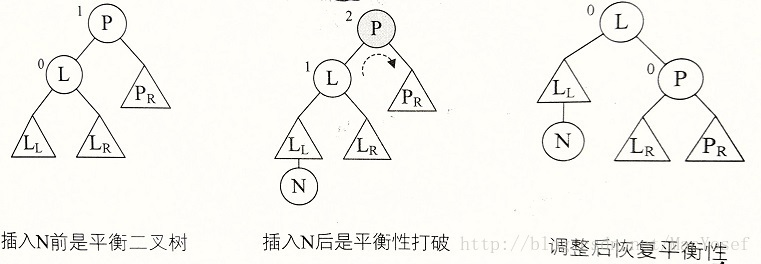• 平衡二叉树，是一种二叉排序树，其中每一个节点的左子树和和右子树的高度差至多等于1。
• 平衡因子，将二叉树的左子树深度减去右子树深度的值称为平衡因子BF（Balance Factor）。
• 最小不平衡子树，距离插入结点最近的，且平衡因子的绝对值大于1的结点为根的子树。

## 平衡二叉树 构建

​平衡二叉树构建的基本思想就是在构建二叉排序树的过程中，每当插人一个结点时，先检查是否因插入而破坏了树的平衡性，若是，则找出最小不平衡子树，在保持二叉排序树特性的前提下，调整最小不平衡子树中各结点间的链接关系，进行相应的旋转，使之成为新的平衡子树。

a={3,2,1,4,5,6,7,10,9,8} 的构建过程

# 主要算法：

/*二叉树的二叉链表结点结构定义*/
typedef struct BitNode				   /*结点结构*/
{
int data;                          /*结点数据*/
int bf;                            /*结点的平衡因子*/
struct BitNode *lchild,*rchild;    /*左右孩子指针*/
}BitNode,*BiTree;

/*对以P为根的二叉树作右旋处理*/
/*处理之后P指向新的树根结点，即旋转处理之前的左子树的根节点*/
void R_Rotate(BiTree *P)
{
BiTree L;
L=(*P)->lchild;
(*P)->lchild = L->rchild;
L->rchild=(*P);
*P =L;
}#define LH 1
#define EH 0
#define RH -1
/*对以指针T所指根节点为根的二叉树作左平衡旋转处理*/
/*本算法结束时，指针T指向新的根节点*/
void LeftBalance(BiTree *T)
{
BiTree L,Lr;
L=(*T)->lchild;    /*L指向T的左子树根节点*/
switch(L->bf)
{    /*检查T的左子树的平衡度，并作相应平衡处理*/
case LH:   /*新结点插入在T的左孩子的左子树上，作右旋处理*/
(*T)->bf=L->bf=EH;
R_Rotate(T);
break;
case RH:   /*新结点插入在T的左孩子的右子树上，作双旋处理*/
Lr = L->rchild;   /*Lr指向T的左孩子的右子树根*/
switch(Lr->bf)    /*修改T及其左孩子的平衡因子*/
{
case LH:
(*T)->bf = RH;
L->bf = EH;
break;
case EH:
(*T)->bf = L->bf = EH;
break;
case RH:
(*T)->bf = EH;
L->bf = LH;
break;
}
Lr->bf = EH;
L_Rotate(&(*T)->lchild);   /*对T的左子树作左旋平衡处理*/
R_Rotate(T);               /*对T作右旋平衡处理*/
break;
}
}


//若在平衡的二叉排序树T中不存在和e有相同关键字的结点，
//则插入一个数据元素为e的新结点并返回1，否则返回0。若因插入而使二叉排序树失去平衡，
//则作平衡旋转处理，布尔变量taller反映T长高与否
Status InsertAVL(BiTree *T,int e,Status *taller)
{
if(!*T)
{   /*插入新结点，树“长高”，置taller为TRUE */
*T=(BiTree)malloc(sizeof(BiTNode));
(*T)->data = e;
(*T)->lchild=(*T)->rchild = NULL;
(*T)->bf=EH;
*taller = TRUE;
}
else
{
if(e==(*T)->data)
{   /*树中已存在和e相同关键字的结点则不再插入*/
*taller=FALSE;
return FALSE;
}
if(e<(*T)->data)
{   /*继续在T的左子树中进行搜索*/
if(!InsertAVL(&(*T)->lchild,e,taller))
return FALSE;
if(*taller) /*已插入到T的左子树中且左子树高度增加*/
{
switch((*T)->bf)   /*检查T的平衡度*/
{
case LH:  /*原本左子树比右子树高，需要作平衡处理*/
LeftBalance(T);
*taller=FALSE;
break;
case EH:  /*原本左右子树等高，现因左子树增高而树增高*/
(*T)->bf=LH;
*taller = TRUE;
break;
case RH:  /*原本右子树高，现左右子树等高*/
(*T)->bf = EH;
*taller = FALSE;
break;
}
}
}
else
{   /*继续在T的左子树中进行搜索*/
if(!InsertAVL(&(*T)->rchild,e,taller))
return FALSE;
if(*taller)
switch((*T)->bf)
{
case LH:
(*T)->bf=EH;
*taller = FALSE;
break;
case EH:
(*T)->bf=RH;
*taller = TRUE;
break;
case LH:
RightBalance(T);
*taller = FALSE;
break;
}
}
}
}
return TRUE;
}